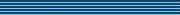### Large deviation theory and its applications in statistical mechanics

Speaker
Dr. H. Touchette
Duration
54:21
Date
24 March 2010
Abstract
The theory of large deviations, initiated by Cramer in the 1930s and later developed by Donsker and Varadhan in the 1970s, is an active field of probability theory that finds applications in many subjects, including statistics, finance, actuarial mathematics, engineering, and physics. Its use in physics dates back to the work of Ruelle, Lanford, and the late John Lewis, among others, who used concepts of large deviations in the 1970s and 1980s to study equilibrium systems and to put statistical mechanics on a rigorous footing.

I will give in this talk a survey of these applications, as well as more recent ones related to long-range equilibrium systems and nonequilibrium systems, at a level which assumes little knowledge of statistical mechanics or large deviations. As we cover these applications, we will see that large deviation theory and statistical mechanics share a common mathematical structure, which Lewis was well aware of, and which can be summarized by saying that an entropy function is to a physicist what a large deviation function (or rate function) is to a mathematician. Other connections of this sort will be discussed.
You should see the video here…
High quality
Fullscreen
Volume